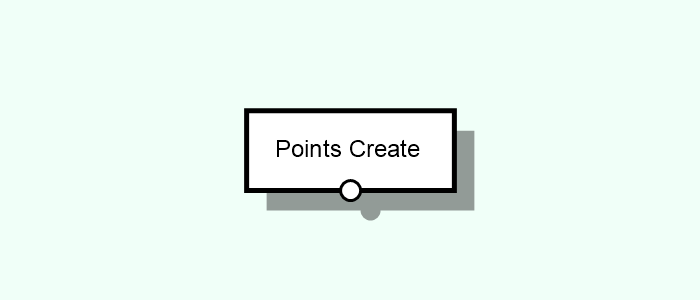# Points Create

Creates points from primitive patterns (grid, random) or from other Entity types.Tags: Point, From Mesh, From Path, From Surface, Grid, Random, Simple

## Parameters#

Type [Select]
Type/style of points to create.
From Mesh [Compound] [Adds ports 1]
Extracts points from mesh vertices. Shared vertices are not repeated.
From Path [Compound] [Adds ports 2]
Extracts points from path vertices. Shared vertices are not repeated.
From Surface [Compound] [Adds ports 3]
Extracts points from surface vertices.
Coordinates [Attribute]
Attribute to store the surface-relative coordinates (column/row) of the vertices that originated the point.
Grid [Compound]
Creates a 3D grid of points.
Columns [Int]
Number of points in the X direction.
Rows [Int]
Number of points in the Y direction.
Layers [Int]
Number of points in the Z direction.
Spacing [Vector3D]
The spacing in the X,Y,Z dimensions between each point.
Random [Compound]
Creates randomly scattered points.
Seed [Int]
The seed of the random point distribution.
Area [Select]
Area on which the points are to be distributed.
Mesh [Compound] [Adds ports 4, 5]
Scatters points on a mesh surface.
Distance [Float]
The (minimum) distance between the points.
In practice, the value will vary between distance and 2 x distance.
Rectangle [Compound]
Scatters points within a 2D rectangular area.
Size [Vector2D]
The size of the area where to scatter the points.
Distance [Float]
The (minimum) distance between the points.
In practice, the value will vary between distance and 2 x distance.
Surface [Compound] [Adds ports 6, 7]
Scatters points on a surface.
Distance [Select]
The (minimum) distance between the points.
In practice, the value will vary between distance and 2 x distance.
Fixed [Compound]
Sets a fixed distance between the points.
Distance [Float]
The (minimum) distance between the points.
In practice, the value will vary between distance and 2 x distance.
Layer [Compound]
Sets a variable distance between the points, based on the intensity of a chosen layer. The intensity values of the layer will be mapped to the generated point density. Layer values below a certain threshold will be filtered so as to define areas where no points are generated.
Index [Int]
Index of the layer which contains the intensity information. Should be a floating-point based layer, such as a height or blend layer.
By default, the index 0 means the height layer.
Min. Threshold [Float]
The layer value below which no points will be placed (in a 0-1 relative range). This works as a mask, meaning that, for instance, in those areas where the layer value is below this value, no points will be placed.
Min. Distance [Float]
The minimum distance between the generated points. This corresponds to the layer areas with highest values, creating therefore areas with higher point density.
Max. Distance [Float]
The maximum distance between the generated points. This corresponds to the layer areas with lowest values, creating therefore areas with lower point density.
Distance [Attribute]
The actual distance calculated for the point.
Simple [Compound]
Creates points from a list of 3D locations.
Points [List]
List of locations of the points.
Point [Vector3D]
Location of the point.

## Inputs#

This node has no native inputs.

### Parameter Inputs#

•  Mesh [Single | Mesh]: The mesh from which the points are to be extracted from.
•  Path [Single | Path]: The path from which the points are to be extracted from.
•  Surface [Single | Surface]: The surface from which the points are to be extracted from.
•  Mesh [Single | Mesh]: The mesh on which to randomly scatter the points.
•  Surface [Single | Surface]: The surface on which to randomly scatter the points.

## Outputs#

• Output [Point]: Points created according to the defined parameters and/or inputs.

### Parameter Outputs#

•  Mesh [Mesh]: The mesh on which the points were scattered.
•  Surface [Surface]: The surface on which the points were scattered.